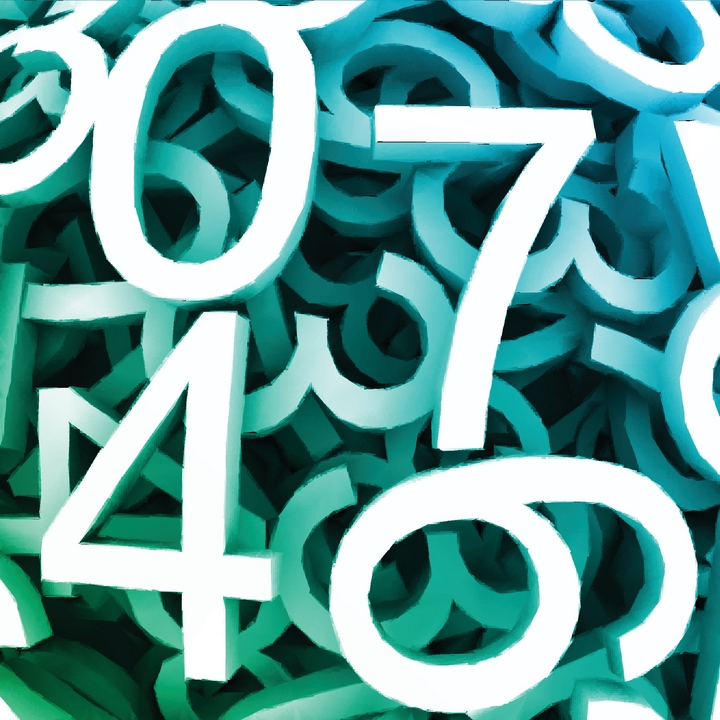# The Final Number

Algebra Level 3Your Math Prof asks you to write down the Numbers from 1 to 60.

He says, "Add the first two numbers, and place this new number at the end, then erase the first two numbers.

For example, after the first time, the numbers become 3, 4, 5, ..., 59, 60, and 3.

Repeat this process until you get only one number. Whats that last number after you have completed the entire process?"

The other students start the tedious procedure but you are of course smarter than them!

Can you quickly tell your Math Prof what would be The Final Number?

×